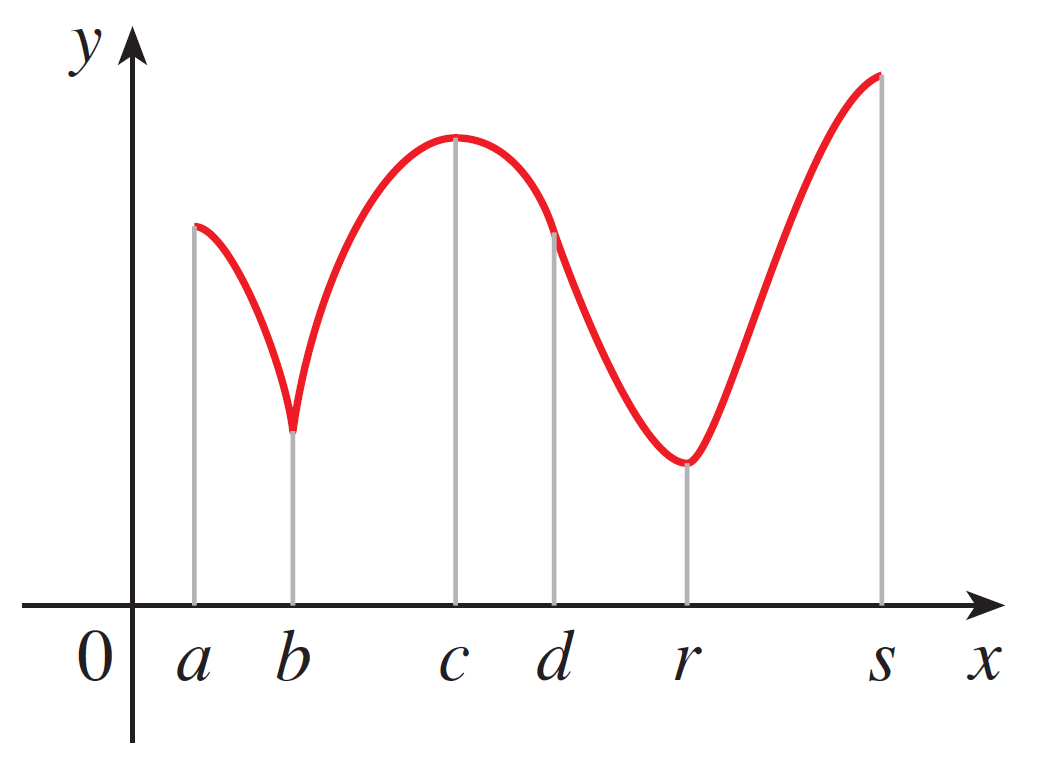# Homework 8

Directions:

1. Show each step of your work and fully simplify each expression.
2. Turn in your answers in class on a physical piece of paper.
3. Staple multiple sheets together.
4. Feel free to use Desmos for graphing.

1. Find the Taylor polynomial of degree 3 at $a = 0$ for $f(x) = \sin \pi x$.
2. Find the Taylor polynomial of degree 4 at $a = 1$ for $f(x) = \dfrac{1}{x}$.
3. Explain the difference between an absolute minimum and a local minimum.
4. If $f$ is a continuous function on $(a, b)$, does an absolute maximum have to exist?
5. Identify all absolute/local minimums and maximums for the following function:6. Draw a graph that is continuous on $[-2, 5]$ and has absolute minimum at $x = 3$, absolute maximum at $x = 0$.
7. Find the critical numbers for the following functions:
1. $f(x) = x^3 + 6x^2 - 15x$
2. $f(x) = x^2e^{-3x}$
8. Find the absolute maximum and minimum for the following functions:
1. $f(x) = 12 + 4x - x^2, \qquad [0, 5]$
2. $f(x) = x - \ln x, \qquad \left[\frac{1}{2}, 2\right]$
9. Find the intervals on which $f$ is increasing and all local minimum and maximum values:
1. $f(x) = 2x^3 + 3x^2 - 36x$
2. $f(x) = x^2e^{-3x}$
3. $f(x) = \dfrac{\ln x}{\sqrt{x}}$
10. Suppose $f(x)$ is not continuous and you use the First Derivative Test to find all local minimums and maximums. Draw a picture of the situation where a found local maximum isn't actually a real one.
11. Why does the Increasing/Decreasing Test work?